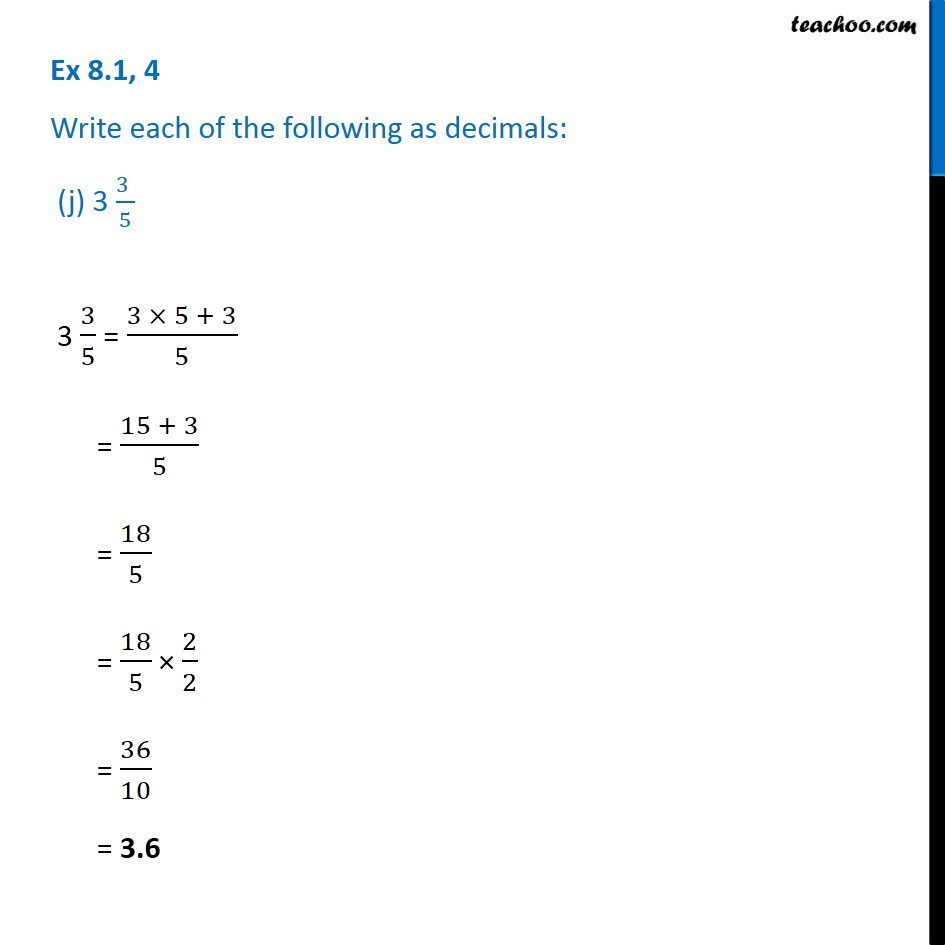1. Chapter 8 Class 6 Decimals
2. Serial order wise
3. Ex 8.1

Transcript

Ex 8.1, 4 Write each of the following as decimals: (j) 3 (3 )/5 3 3/5 = (3 × 5 + 3)/5 = (15 + 3)/5 = 18/5 = 18/5 × 2/2 = 36/10 = 3.6